Question

# For a Carnot engine with 10 moles of ideal gas (Cv = 1.5 nR) and operating...

For a Carnot engine with 10 moles of ideal gas (Cv = 1.5 nR) and operating between a hot reservoir of 500 K and a cold reservoir of 300 K,

a) What would be the heat exchanges (q1) and entropy change (∆S1) for step 1, where the gas reversibly and isothermally expands to double its volume (V2 = 2 V1) at 500 K?

b) What would be the heat exchanges (q3) and entropy change (∆S3) for step 3, where the gas is reversibly and isothermally compressed to half its volume (V4 = 0.5 V3) at 300 K?

c) What would be the net work done by this Carnot engine within each cycle?

d) What would be the efficiency of this Carnot engine?

Thank you!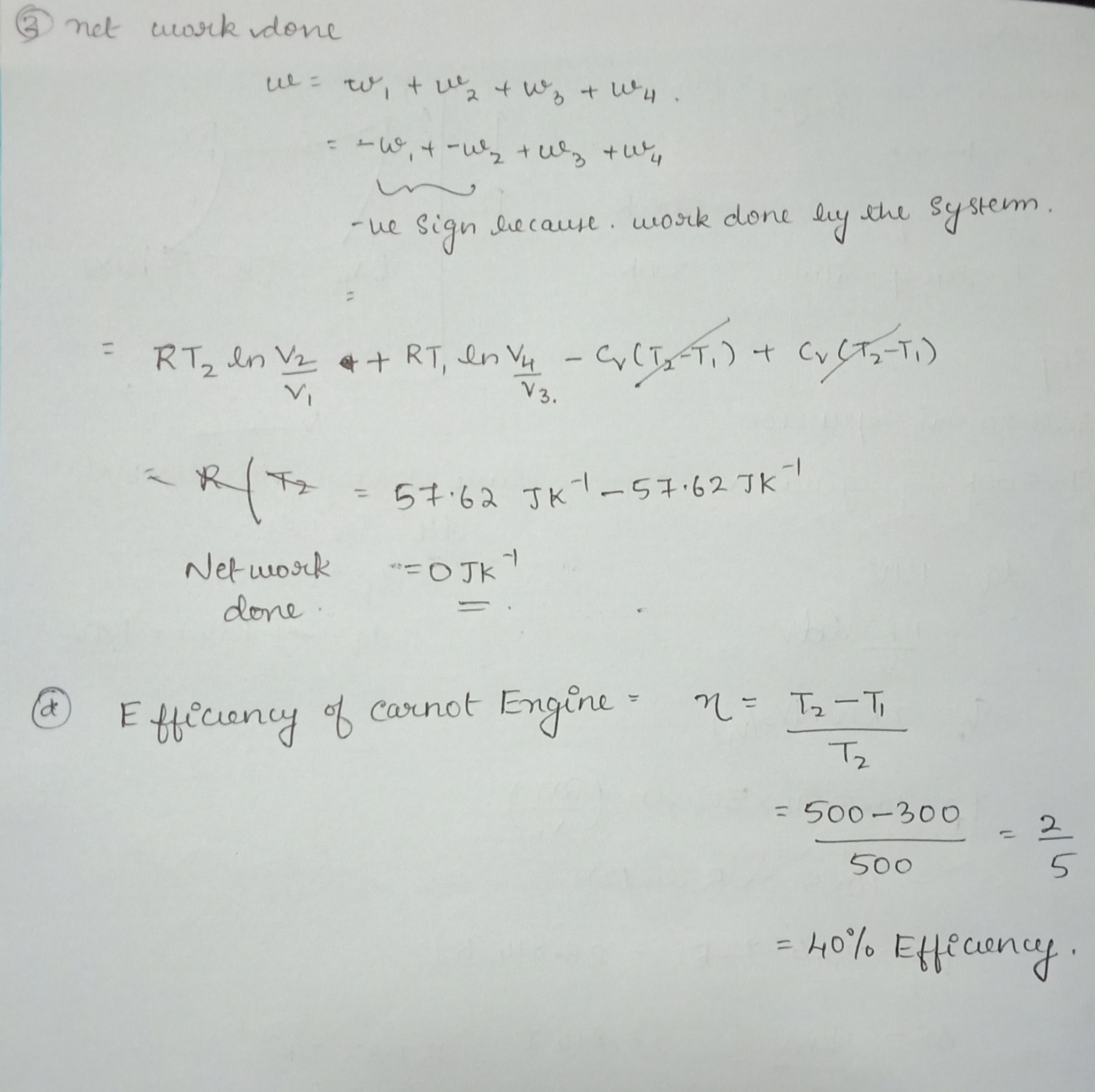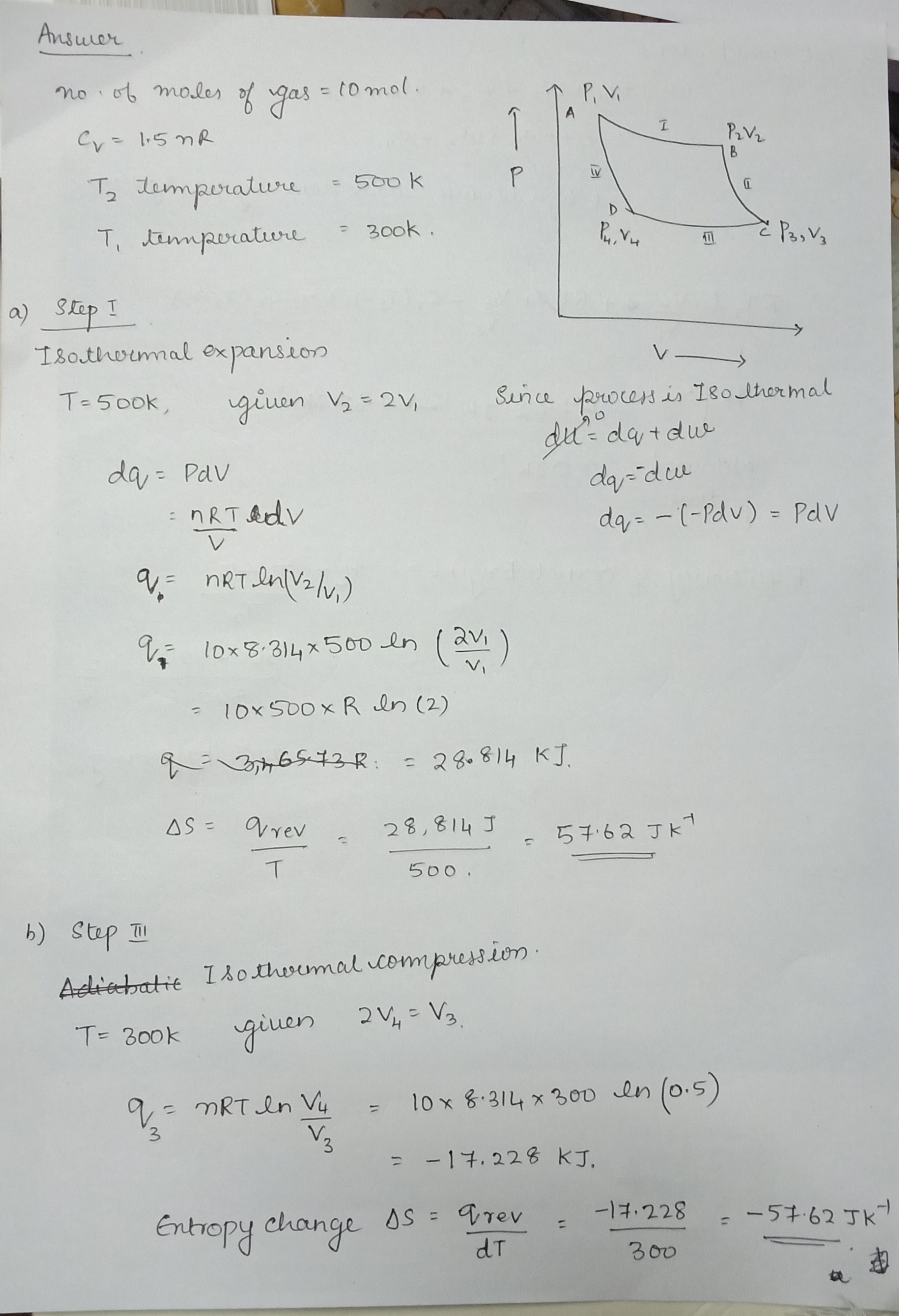#### Earn Coins

Coins can be redeemed for fabulous gifts.

Similar Homework Help Questions
• ### For a Carnot engine with 10 moles of ideal gas (Cv= 1.5 nR) and operatingbetween a...

For a Carnot engine with 10 moles of ideal gas (Cv= 1.5 nR) and operatingbetween a hot reservoir of 500 K and a cold reservoir of 300 K,a. (6 Points) What would be the heat exchanges (q1) and entropy change (∆S1) for step 1, where thegas reversibly and isothermally expands to double its volume (V2= 2 V1) at 500 K?b. (6 Points) What would be the heat exchanges (q3) and entropy change (∆S3) for step 3, where thegas is reversibly...

• ### A Carnot engine operates us ing 1.0 mol e of monoatomic ideal gas as a working...

A Carnot engine operates us ing 1.0 mol e of monoatomic ideal gas as a working s ubstance. In t he first step, the gas is place d in thermal contact with a heat reservoir and expands isothermally to 3 .0 times its initial volume. (a) If the internal energy o f the gas after this step is 6.25 k J , calculate the temperature of the heat reservoir ( T h ) . (b) C alculate the heat absorbed...

• ### 1. A Carnot engine using 2 moles of ideal diatomic gas is operating between 625 C...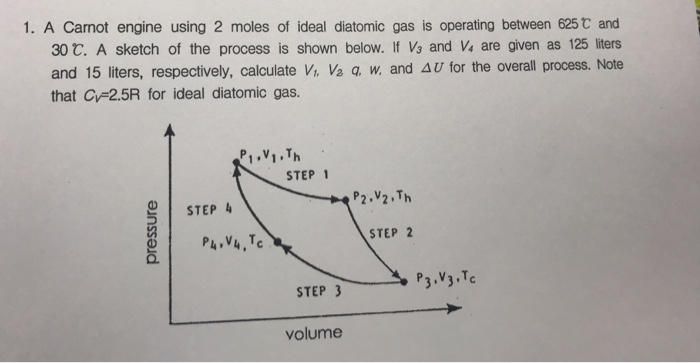1. A Carnot engine using 2 moles of ideal diatomic gas is operating between 625 C and 30 C. A sketch of the process is shown below. If Vs and V4 are given as 125 liters and 15 liters, respectively, calculate V. Va W, and Δ U for the overall process. Note that Cv-2.5R for ideal diatomic gas. P.VI.Th STEP 1 STEP 4 STEP 2 volume

• ### 69. A Carnot engine employs 1.5 mol of nitrogen gas as a working substance, which is...

69. A Carnot engine employs 1.5 mol of nitrogen gas as a working substance, which is considered as an ideal diatomic gas with γ =7.5 at the working temperatures of the engine. The Carnot cycle goes in the cycle ABCDA with AB being an isothermal expansion. The volume at points A and C of the cycle are 5.0×10−3 m3 and 0.15 L, respectively. The engine operates between two thermal baths of temperature 500 K and 300 K. (a) Find the...

• ### Two moles of an ideal gas occupy a volume V. The gas expands isothermally and reversibly...

Two moles of an ideal gas occupy a volume V. The gas expands isothermally and reversibly to a volume 6 V. A)Is the velocity distribution changed by the isothermal expansion? B)Explain why ? C)Use the equation ΔS=klnw2/w1 to calculate the change in entropy of the gas. D)Use the equation ΔS=Q/T to calculate the change in entropy of the gas. Compare the result in part (d) to that obtained in part (c).

• ### 102) 2.37 moles of an ideal monatomic gas initially at 255 K undergoes this cycle: It...

102) 2.37 moles of an ideal monatomic gas initially at 255 K undergoes this cycle: It is (1) heated at constant pressure to 655 K, (2) then allowed to cool at constant volume until its temperature returns to its initial value, (3) then compressed isothermally to its initial state. Find: a. the net energy transferred as heat to the gas (excluding the energy transferred as heat out of the gas). b. the net work done by the gas for the...

• ### 1.3 mole of an ideal gas at 300 K is expanded isothermally and reversibly from a...

1.3 mole of an ideal gas at 300 K is expanded isothermally and reversibly from a volume V to volume 4V. What is the change in entropy of the gas, in J/K?

• ### Problem 6. Why do you think Carnot engine is the most efficient engine? Choose all correct...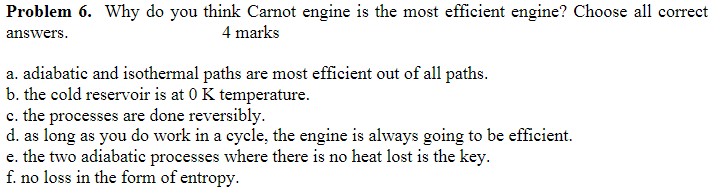Problem 6. Why do you think Carnot engine is the most efficient engine? Choose all correct answers. 4 marks a. adiabatic and isothermal paths are most efficient out of all paths. b. the cold reservoir is at 0 K temperature. c. the processes are done reversibly. d. as long as you do work in a cycle, the engine is always going to be efficient. e. the two adiabatic processes where there is no heat lost is the key. f. no...

• ### A 2.5 mole sample of an ideal gas expands reversibly and isothermally at 360 K until...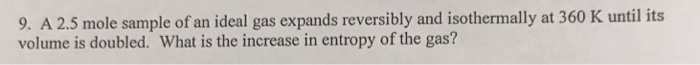A 2.5 mole sample of an ideal gas expands reversibly and isothermally at 360 K until its volume is doubled. What is the increase in entropy of the gas?

• ### If the engine uses 5 moles of an ideal gas what are the volumes: V1, V2,...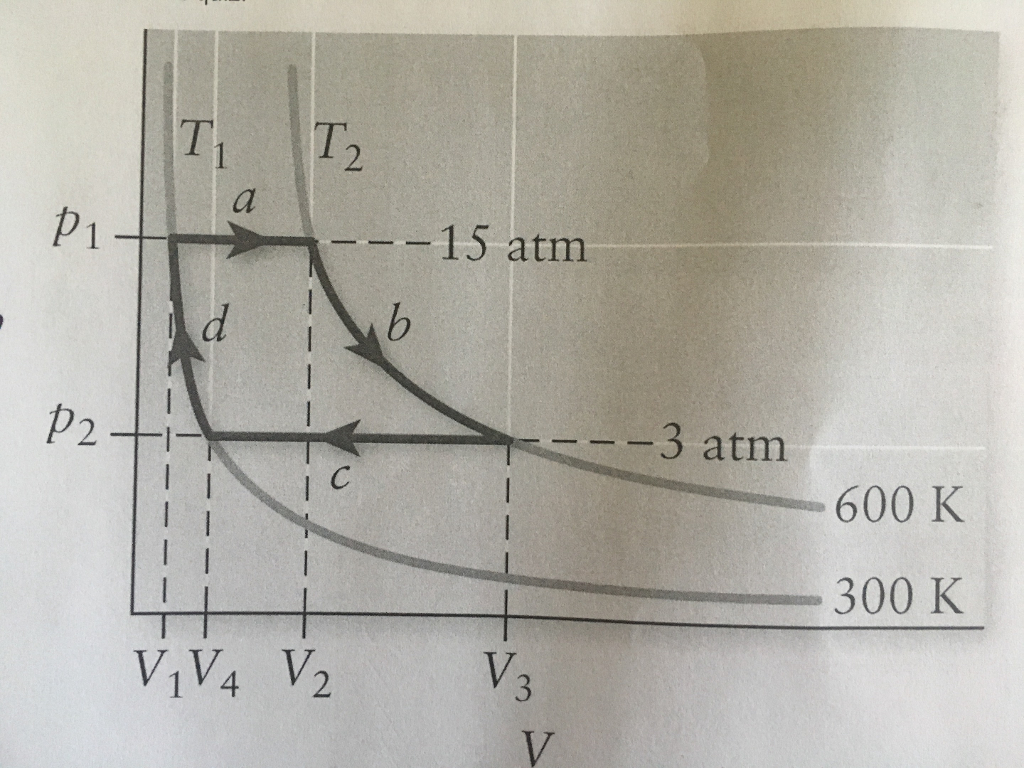If the engine uses 5 moles of an ideal gas what are the volumes: V1, V2, V3, and V4? T, IT, p1 15 atm p2 3 atm 600 K 300 K 1V4 V2